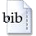User: GuestLogin
Document type:
Technical Report
Author(s):
Ernst W. Mayr
Title:
Scheduling Interval Orders in Parallel
Abstract:
Interval orders are partial orders defined by having interval representations. It is well known that a transitively oriented digraph G is an interval order iff its (undirected) complement G- is chordal. We investigate parallel algorithms for the following scheduling problem: Given a system consisting of a set T of n tasks (each requiring unit execution time) and an interval order < over T, and given m identical parallel processors, construct an optimal (i.e., minimal length) schedule for (T,<). Our algorithm is based on a subroutine for computing so-called scheduling distances, i.e., the minimal number of time steps needed to schedule all those tasks succeeding some given task t and preceding some other task t-. For a given interval order with n tasks, these scheduling distances can be computed using n^3 processors and O(log^2n) time on a CREW-PRAM. We then give an incremental version of the scheduling distance algorithm, which can be used to compute the empty slots in an optimal schedule. From these, we derive the optimal schedule, using no more resources than for the initial scheduling distance computation and considerably improving on previous work by Sunder and He. The algorithm can also be extended to handle task systems which, in addition to interval order precedence constraints, have individual deadlines and/or release times for the tasks. Our algorithm is the first NC-algorithmfor this problem. As another application, it also provides NC-algorithms for some graph problems on interval graphs, (which are NP-completein general).
Keywords:
Intervall orders; scheduling problem.
Year:
1994
Year / month:
1994-05-00 00:00:00
Pages:
15BibTeX